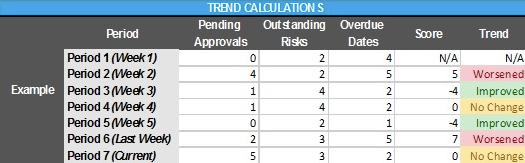1. Navigate Risk for Escalation

From the ‘Project Overview’ page, scroll to the ‘Risk for Escalation’ section.

To see the Risk Trend, hover over ‘Trend’ at the top right corner of the section.Note: Overview page is only for viewing. Risks for Escalation can be managed via Risks page.

Risks for Escalation > Trend

• Trend Period: A ‘‘Trend Period’ is equal to 1 calendar week
• Trend History: Displays the previous 4 periods of the trend
• Current Trend Update Frequency: Every update, edit, addition, etc. to the Budget section within the current trend period

Trend Icon:

• Improved: If the sum of the below three calculations equal a ‘negative’ (-):
• Count of Risks ‘Pending Approval’ compared to Last Period (E.g. two less = -2, or one more = +1)
• Count of Risks that the stage is either ‘Identified’, ‘Analysis Complete’, ‘Planning Complete’ or ‘Actions in Implementation’ compared to Last Period (E.g. two less = -2, or one more = +1)
• Count of Risks past their ‘Resolution Date’ compared to Last Period (E.g. two less = -2, or one more = +1

• No Change: If the sum of the below three calculations equal ‘0’:
• Count of Risks ‘Pending Approval’ compared to Last Period (E.g. two less = -2, or one more = +1)
• Count of Risks that the stage is either ‘Identified’, ‘Analysis Complete’, ‘Planning Complete’ or ‘Actions in Implementation’ compared to Last Period (E.g. two less = -2, or one more = +1)
• Count of Risks past their ‘Resolution Date’ compared to Last Period (E.g. two less = -2, or one more = +1)

• Worsened: If the sum of the below three calculations equal a ‘positive’ (+):
• Count of Risks ‘Pending Approval’ compared to Last Period (E.g. two less = -2, or one more = +1)
• Count of Risks that the stage is either ‘Identified’, ‘Analysis Complete’, ‘Planning Complete’ or ‘Actions in Implementation’ compared to Last Period (E.g. two less = -2, or one more = +1)
• Count of Risks past their ‘Resolution Date’ compared to Last Period (E.g. two less = -2, or one more = +1)

Example Risk Calculations: